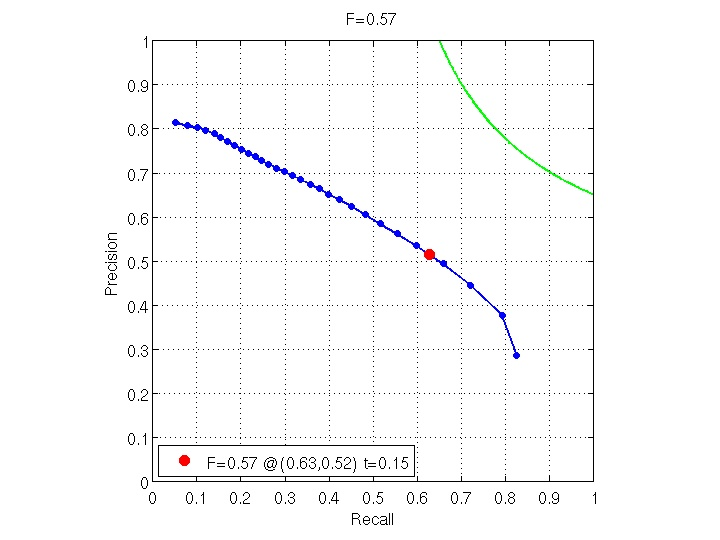## [Grayscale] Boundary Detection Benchmark: Algorithm "Second Moment Matrix"

This algorithm finds boundaries by examining the eigenspectrum of the spatially averaged second moment matrix. Image gradients are estimated with a pair of Gaussian derivative filters. The two eigenvalues are used as features in a logistic model to estimate the posterior probability of a boundary at each pixel. For these 480x320 pixel images, the optimal scale is sigma=2 (for both inner and outer scales). If we order the eigenvalues from smaller to larger, the logistic coefficients are -7.33 and 30.9, with an offset of -3.25.Click on an image for additional details.

Page generated on 20-Feb-2013 11:08:18.